# Top 10 New Features Of C# 7

Most of you are already aware that Visual Studio 2017 RC has been released and it comes with a lot of new features. In this article, I am going to discuss only about C# 7 features. The other features will be explained in my upcoming articles.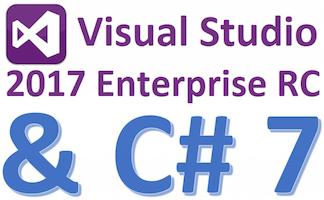In C# 7, a lot of new features have been introduced, and also enhancement have been done for some features. I will be covering all those things in context of Visual Studio 2017 RC. Given below is the list of features which I am going to explain in this article.

1. Local functions or nested functions
2. Binary Literal
3. Digit Separators
4. Pattern matching
5. ref returns and ref Locals
6. Tuples (Tuples Enhancement)
7. Throw Expressions
8. Expression Bodied Members (some more expression bodied members in C# 7)

1. Expression bodied Methods (C# 6.0)
2. Expression bodied Properties (C# 6.0)
3. Expression bodied constructor (C# 7.0)
4. Expression bodied destructor (C# 7.0)
5. Expression bodied getters (C# 7.0)
6. Expression bodied setters (C# 7.0)

9. Out variables (enhancement for out variables)
10. Deconstruction

In the above list, I have mentioned only 10 new features of C# 7 but there are some more features like Generalized async (Generalized async return), Replace (Partial Class Enhancements) Records (or Record Types) Non-Nullable Reference Types, Immutable Types etc.

Some of the features are not yet supported in Visual Studio 2017 RC and are still under development. Even C# 7 has not been developed completely and still it is in preview mode. You can ignore some of the features of C# 7 right now and focus on the features which can be tested in Visual Studio 2017 RC. Below is a pictorial representation of the major features of C# 7 which are supported in Visual Studio 2017 RC.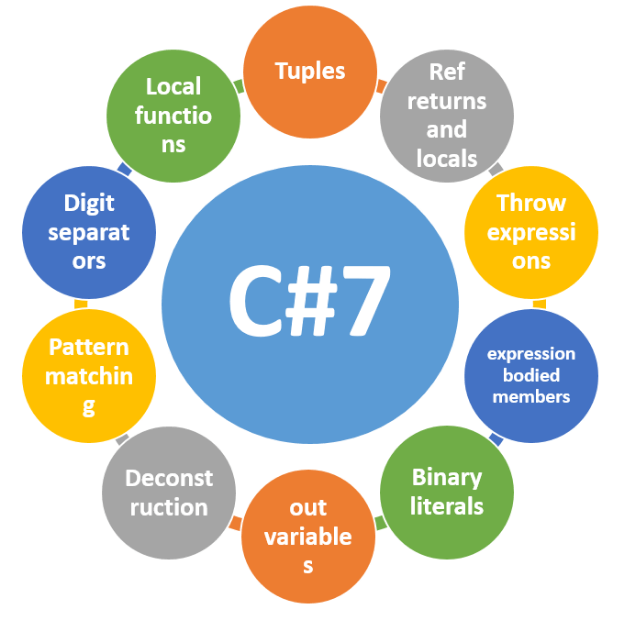1. Local functions or nested functions

Using nested functions inside a function is called local function. To understand how local function works, just have a look at the below code and see how you are going to write and call Add() and Multiply() methods.

Example 1
1. using static System.Console;
2. namespace UseLocalFunctions {
3.     class Program {
4.         static void Main(string[] args) {
5.             void Add(int x, int y) {
6.                 WriteLine(\$ "Sum of {x} and {y} is : {x + y}");
7.             }
8.             void Multiply(int x, int y) {
9.                 WriteLine(\$ "Multiply of {x} and {y} is : {x * y}");
11.             }
13.             Multiply(20, 50);
14.         }
15.     }
16. }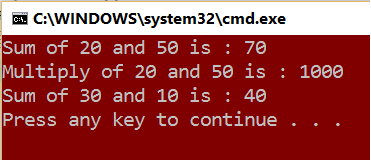In the above example, I have used 2 nested functions “Add” & “Multiply” and you can see that those can be called from anywhere inside the parent function even though I have called inline function “Add” inside another inline function Multiply.

Example 2

In the first example, you have seen that nested function is returning void but there are no such restrictions and it can also return any other type. In this example, I am going to create a local function “CalculateMarks” which will calculate total marks and return it as decimal datatype.

1. public static void PrintStudentMarks(int studentId, params Subject[] subjects) {
2.     WriteLine(\$ "Student Id {studentId} Total Marks: {CalculateMarks()}");
3.     WriteLine(\$ "Subject wise marks");
4.     foreach(var subject in subjects) {
5.         WriteLine(\$ "Subject Name: {subject.SubjectName} \t Marks: {subject.Marks}");
6.     }
7.     decimal CalculateMarks() {
8.         decimal totalMarks = 0;
9.         foreach(var subject in subjects) {
10.             totalMarks += subject.Marks;
11.         }
12.         return totalMarks;
13.     }
14. }

Complete code

1. class Program {
2.     static void Main(string[] args) {
3.         PrintStudentMarks(101, new Subject {
4.             SubjectName = "Math", Marks = 96
5.         }, new Subject {
6.             SubjectName = "physics", Marks = 88
7.         }, new Subject {
8.             SubjectName = "Chem", Marks = 91
9.         });
10.     }
11.     public static void PrintStudentMarks(int studentId, params Subject[] subjects) {
12.         WriteLine(\$ "Student Id {studentId} Total Marks: {CalculateMarks()}");
13.         WriteLine(\$ "Subject wise marks");
14.         foreach(var subject in subjects) {
15.             WriteLine(\$ "Subject Name: {subject.SubjectName} \t Marks: {subject.Marks}");
16.         }
17.         decimal CalculateMarks() {
18.             decimal totalMarks = 0;
19.             foreach(var subject in subjects) {
20.                 totalMarks += subject.Marks;
21.             }
22.             return totalMarks;
23.         }
24.     }
25.     public class Subject {
26.         public string SubjectName {
27.             get;
28.             set;
29.         }
30.         public decimal Marks {
31.             get;
32.             set;
33.         }
34.     }
35. }

Output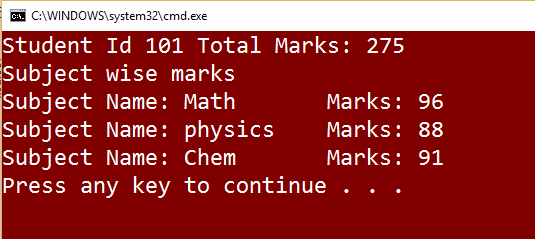Example 3

So far, I have explained the use of local function for printing some values or for returning a calculated variable from local function but apart from that, you can do a lot more. In this example, I am going to explain how we can use local function instead of recursive function.

Calculate factorial with recursive function.

1. private static long GetFactorial(int number) {
2.     if (number < 0) throw new ArgumentException("negative number", nameof(number));
3.     if (number == 0) return 1;
4.     return number * GetFactorial(number - 1);
5. }

Calculate factorial with help of local function

1. private static long GetFactorialUsingLocal(int number) {
2.     if (number < 0) throw new ArgumentException("negative number", nameof(number));
3.     if (number == 0) return 1;
4.     long result = number;
5.     while (number > 1) {
6.         Multiply(number - 1);
7.         number--;
8.     }
9.     void Multiply(int x) => result *= x;
10.     return result;
11. }

I know that most of the readers are aware that it is not necessary to call recursive function to calculate factorial . You may be thinking that if the factorial can also be calculated without using recursive function, then why I am discussing about recursive function. I am discussing recursive function because I want to compare recursive function with local function, and suggesting you replace the recursive function with local function whenever performance matters.

Local function does not need to maintain call stack where recursive function maintains call stack.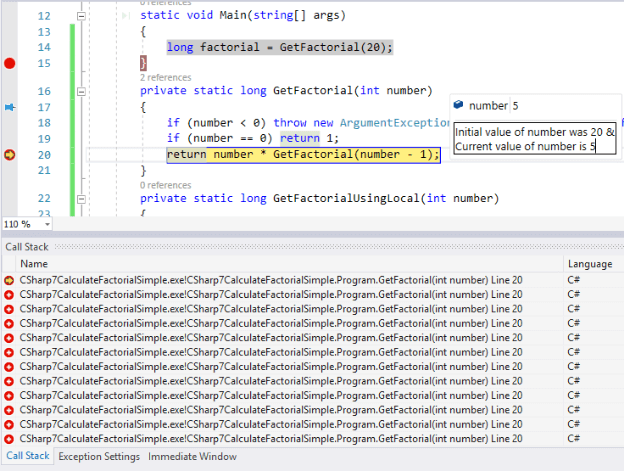Image: Screenshot of call stack maintained by recursive function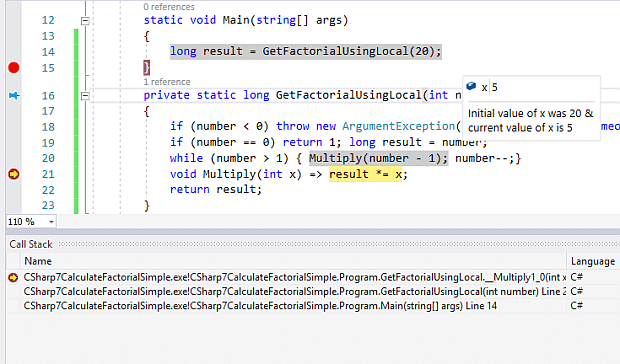Image: Screenshot of call stack maintained by local function

So, in the above screenshot, you can see that no extra call stack has been maintained.

I want to mention one more thing for new readers (who are not aware about call stack limitation). If your call stack is too long, your application will fail. Let’s try the same application for calculation of factorial of 9K.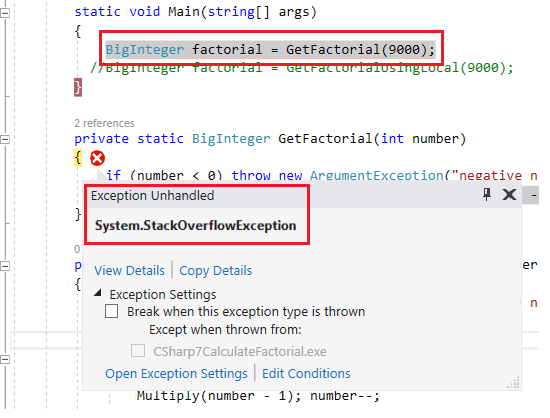You can see that the recursive function is throwing “System.StackOverflowException” exception and application has crashed.

Now, call local method to calculate the factorial of 9k.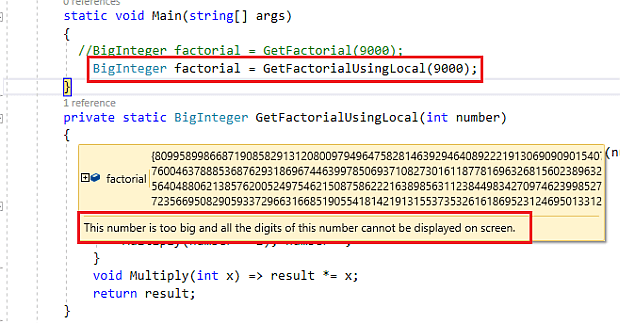Below is the complete code for the same.

1. class Program {
2.     static void Main(string[] args) {
3.         //BigInteger factorial = GetFactorial(9000);
4.         BigInteger factorial = GetFactorialUsingLocal(9000);
5.     }
6.     private static BigInteger GetFactorialUsingLocal(int number) {
7.         if (number < 0) throw new ArgumentException("negative number", nameof(number));
8.         else if (number == 0) return 1;
9.         BigInteger result = number;
10.         while (number > 1) {
11.             Multiply(number - 1);
12.             number--;
13.         }
14.         void Multiply(int x) => result *= x;
15.         return result;
16.     }
17.     private static BigInteger GetFactorial(int number) {
18.         if (number < 0) throw new ArgumentException("negative number", nameof(number));
19.         return number == 0 ? 1 : number * GetFactorial(number - 1);
20.     }
21. }

You can do more experiments on local functions.

2. Binary Literals

As you know that before C# 7, two types of notations were supported in C# - decimal literal and hexadecimal literal.

Decimal Literal

int length = 50;

int number = 0X3E8;
or
int number = 0x3E8;

So, you can see that when you are not using any prefix with integer data type, then it is by default denoting a number in decimal system whereas for a hexadecimal number, we need to use prefix “0X” or “0x” (you can use either small ‘x’ or capital ‘X’).

But, when you print it using Console.WriteLine(), then it will always print it in decimal value. Below is an example for the same.

1. int Int32MaxLengthInDecimal = 2147483647;
3. WriteLine(\$ "Int32MaxLengthInDecimal {Int32MaxLengthInDecimal}");

Output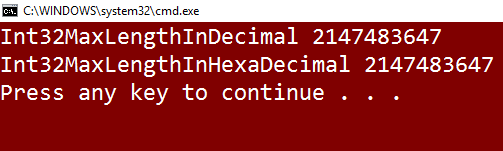In C# 7, the same way, you can also declare a binary literal. You can use “0B” or “0b” for binary literal. So, in C# 7, you can represent integer in 3 ways - decimal literal, hexadecimal literal, and binary literal.

1. Binary Literal
2. int x = 0B110010;
3. or
4. int x = 0b110010;
5. using static System.Console;
6. namespace BinaryLiteral {
7.     class Program {
8.         static void Main(string[] args) {
9.             //Represent 50 in decimal, hexadecimal & binary
10.             int a = 50; // decimal representation of 50
11.             int b = 0X32; // hexadecimal representation of 50
12.             int c = 0B110010; //binary representation of 50
13.             //Represent 100 in decimal, hexadecimal & binary
14.             int d = 50 * 2; // decimal represenation of 100
15.             int e = 0x32 * 2; // hexadecimal represenation of 100
16.             int f = 0b110010 * 2; //binary represenation of 100
17.             WriteLine(\$ "a: {a:0000} b: {b:0000} c: {c:0000}");
18.             WriteLine(\$ "d: {d:0000} e: {e:0000} f: {f:0000}");
19.         }
20.     }
21. }

3. Digit Separators

Digit separator is also a new feature in C# 7. We can use one or more than one Underscore( _ ) character for digit separators. Sometimes, it is required when we are going to represent a big number. So, the code snippet would look something like.

1. using static System.Console;
2. namespace DigitSeparators {
3.     class Program {
4.         static void Main(string[] args) {#
5.             region Using Digit Separators
6.             int binaryData = 0B0010 _0111_0001_0000; // binary value of 10000
8.             int decimalData = 104 _567_789;
9.             int myCustomData = 1 ___________2__________3___4____5_____6;
10.             double realdata = 1 _000 .111 _1e1_00;
11.             WriteLine(\$ " binaryData :{binaryData} \n hexaDecimalData: {hexaDecimalData} \n decimalData: {decimalData} \n myCustomData: {myCustomData} \n realdata: {realdata}");#
12.             endregion
13.         }
14.     }
15. }

4. Pattern matching

C# supports the feature of pattern matching. We can do a lot more using this. Below is a simple code snippet.

1. using static System.Console;
2. namespace PatternMatching {
3.     class Program {
4.         static void Main(string[] args) {
5.             //Example 1
6.             var myData = "Custom Data";
7.             var myData2 = myData is string ? "String" : "Not a string";
8.             var myData3 = myData is string a ? a : "Not a String";
9.             WriteLine(myData2);
10.             WriteLine(myData3);
11.             //Example 2
12.             var x = 10;
13.             dynamic y = 0b1001;
14.             var sum = y is int ? \$ "{y * x}" : "Invalid data";
15.             WriteLine(sum);
16.         }
17.     }
18. }

This is a very simple example but pattern matching supports a lot of patterns like Type Pattern, Constant Pattern, Var Pattern, Recursive Pattern, Property Pattern & Property Sub-pattern, Switch Statement, Match Expression, Case expression, Throw expression, Destructuring assignment, Testing Nullable, Arithmetic simplification, Tuple decomposition, Complex Pattern, Wildcard Pattern etc.

5. Out Variables (enhancement for out variables)

Before C# 7, if you had to pass a variable as out parameter, then the variable must had been declared before passing it to the method. But in C# 7, you can declare variable directly when you are passing it inside a method.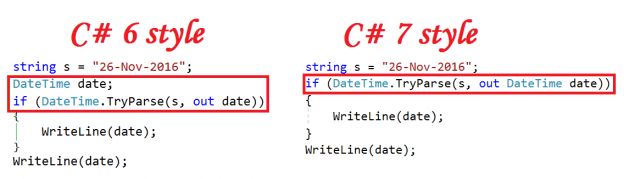In the above screenshot, you can see that for C# 7 I am declaring an out variable inside method and it is being accessed from outside also.

1. using static System.Console;
2. class Program {
3.     static void Main(string[] args) {
4.         string s = "26-Nov-2016";
5.         if (DateTime.TryParse(s, out DateTime date)) {
6.             WriteLine(date);
7.         }
8.         WriteLine(date);
9.     }

You may be thinking that if exception occurs, then what will happen in this case. If any exception occurred in that, the out variable will be assigned with a default value. You can see in the below screenshot that I have passed incorrect date and it has not been converted to DateTime, so a default value has been assigned to variable “date”.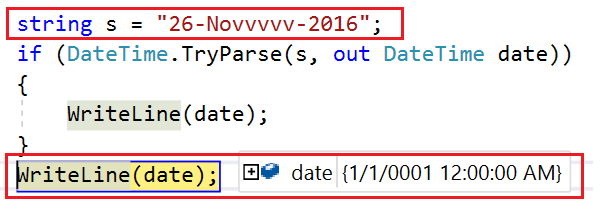6. Tuples

In C# 7, we can use Tuples directly i.e. in more convenient way. Below is a sample code

1. //returning price & discount
2. (intint) GetPrice(int itemId) {
3.     var product = (500, 100);
4.     return product;
5. }

In the above code, I want to pass ItemId as parameter and want two return values - Item price and discount. But, if you would run the above code directly in Visual Studio 2017 RC, then it will not work. To make it work properly, you have to do a bit extra. I am going to explain all those steps one by one here.

Below is a screenshot of error which you will get at the first attempt.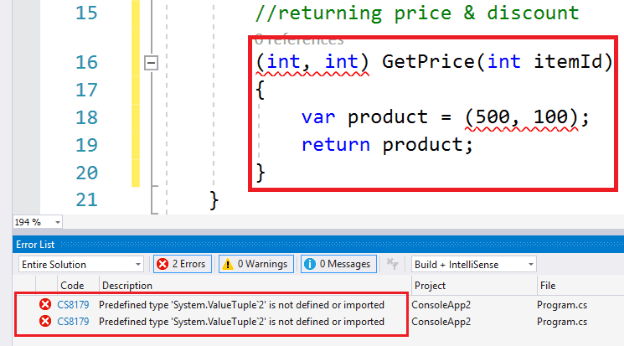Now, move to its solution.

Solution

Install “System.ValueTuple” from NuGet Package.

• Right click on it.
• Select “Manage NuGet Packages…” from context menu.
• Search for “System.ValueTuple” and install it.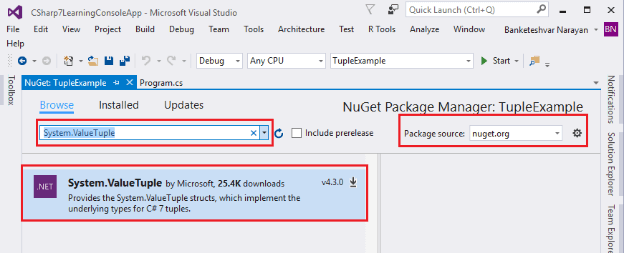Now, re-build your solution. You will notice that error has been removed and your application is built successfully.

Now, you may be thinking how to utilize or call this tuple method. It’s very simple. Just have a look at the below code snippet.

1. using static System.Console;
2. using static System.Text.Encoding;
3. namespace TupleExampleByBNarayan {
4.     class Program {
5.         static void Main(string[] args) {
6.                 OutputEncoding = UTF8;
7.                 Program p = new Program();
8.                 var price = p.GetPrice(1);
9.                 WriteLine(\$ "Price: ₹{price.Item1}/- \nDiscount: ₹{price.Item2}/-");
10.             }
11.             //returning price & discount
12.             (intint) GetPrice(int itemId) {
13.                 var product = (500, 100);
14.                 return product;
15.             }
16.     }
17. }

Output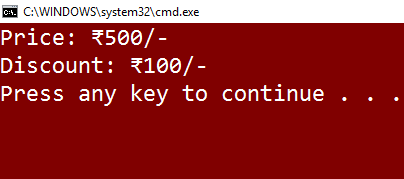But, if you watch the above code snippet closely, you will notice the naming convention issues. I am accessing price with property “Item1” and discount with name “Item2” but it would be better if I have accessed it using their names directly. We can also do that.

Let’s have a look at the below code snippet.

1. using static System.Console;
2. using static System.Text.Encoding;
3. namespace TupleExampleByBNarayan {
4.     class Program {
5.         static void Main(string[] args) {
6.                 OutputEncoding = UTF8;
7.                 Program p = new Program();
8.                 var product = p.GetPrice(1);
9.                 WriteLine(\$ "Price: ₹{product.price}/- \nDiscount: ₹{product.discount}/-");
10.             }
11.             //returning price & discount
12.             (int price, int discount) GetPrice(int itemId) {
13.                 var product = (500, 100);
14.                 return product;
15.             }
16.     }
17. }

You will notice that I have replaced less meaningful names “Item1” & “Item2” with “price” & “discount” respectively.

Internally, you can use any variable name for tuple but externally, it will always return with the same name that is written as return type.

1. (int price, int discount) GetPrice(int itemId) {
2.     var product = (500, 100);
3.     return product;
4. }
5. And(int price, int discount) GetPrice(int itemId) {
6.     var product = (a: 500, b: 100);
7.     return product;
8. }

Both can be accessed by “price” & “discount” irrespective of their internal names. You can do a lot more with tuple. I will explain more about these in coming section and you can also do experiment by yourself.

7. Deconstruction (splitting tuples)

Till now, you have seen that we can access tuples in different ways like accessing with “Item1”, “Item2” or with some meaningful names, like price and discount. Still, we need to access those properties with a collection name like “product.price”. A question must be arising in your mind - can I use the variable name “price” directly without using any other collection name. The answer is obviously YES.

We can say that “deconstruction” is a concept which allows us to access a tuple member directly with its name without using any tuple variable name. Or, you can also say that “deconstruction” is a concept which allows us to split tuple members and we can use it directly with their respective names.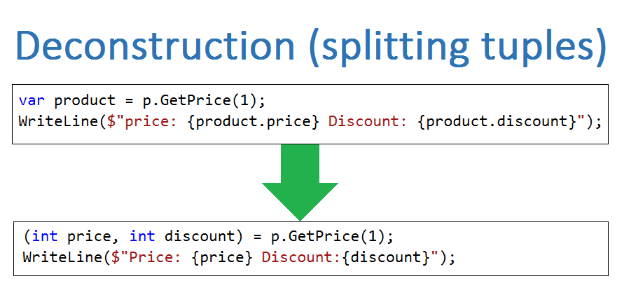8. Expression bodied members

Expression bodied member is not a new concept in C#7. It has been introduced with C#6. But in C#7, some more enhancement has been done for expression bodied members. If you are not aware about expression bodied members, then try to understand it from beginning.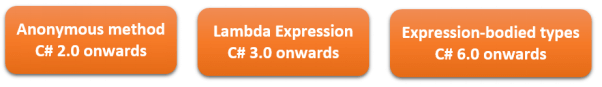Below is a screenshot from my previous article about anonymous methods and lambda expressions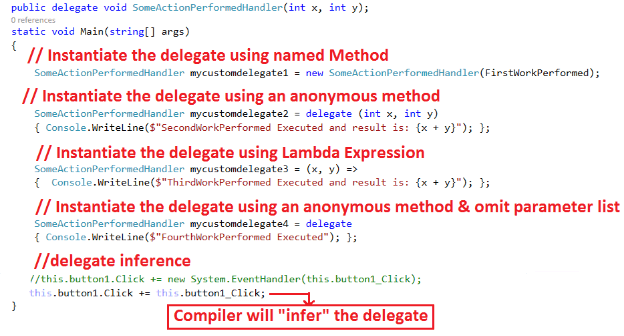If you want to go in depth, then please go through these 2 articles.

C# 6.0

Expression bodied Methods
Expression bodied Properties

C# 7.0

Expression bodied constructor
Expression bodied destructor
Expression bodied getters
Expression bodied setters

Expression Bodied Methods

In C# 6, Microsoft introduced a new feature Expression-bodied Methods which was very similar to and inspired by anonymous & lambda expression. But there are some differences between these two.

In the case of Expression-bodied methods, it must have a return type, name, and returned expression.

We can use access modifiers (private, public, protected, internal and protected internal) with expression-bodied method. We can declare it as virtual, static, or it can also override its parent class method. It can be asynchronous if it is returning void.

Example 1

Expression bodied method has access modifier as public and return type string.Code Snippet

1. class User {
2.     public int UserId {
3.         get;
4.         set;
5.     }
7.         get;
8.         set;
9.     }
10.     public string EmailId {
11.         get;
12.         set;
13.     }
14.     public string ContactNumber {
15.         get;
16.         set;
17.     }
18.     public string GetUserDetails() => \$ "{UserId} + {UserName} + {EmailId} + {ContactNumber}";
19. }

For more details please visit my blog Expression - Bodied Methods in C# 6

Expression Bodied Properties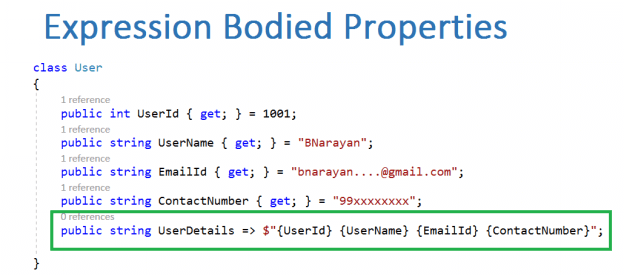Code Snippet

1. class User {
2.     public int UserId {
3.         get;
4.     } = 1001;
6.         get;
7.     } = "BNarayan";
8.     public string EmailId {
9.         get;
10.     } = "bnarayan....@gmail.com";
11.     public string ContactNumber {
12.         get;
13.     } = "99xxxxxxxx";
14.     public string UserDetails => \$ "{UserId} {UserName} {EmailId} {ContactNumber}";
15. }

For more details, please visit my blog

Expression bodied constructor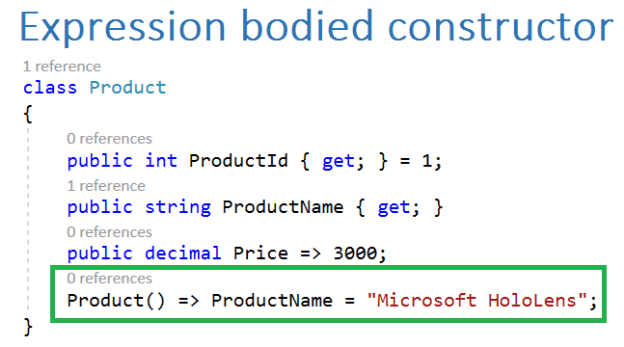In C# 7, we can declare a constructor as “expression bodied members”. Below is a code snippet for the same.

1. class Product {
2.     public int ProductId {
3.         get;
4.     } = 1;
5.     public string ProductName {
6.         get;
7.     }
8.     public decimal Price => 3000;
9.     Product() => ProductName = "Microsoft HoloLens";
10. }

Expression bodied destructor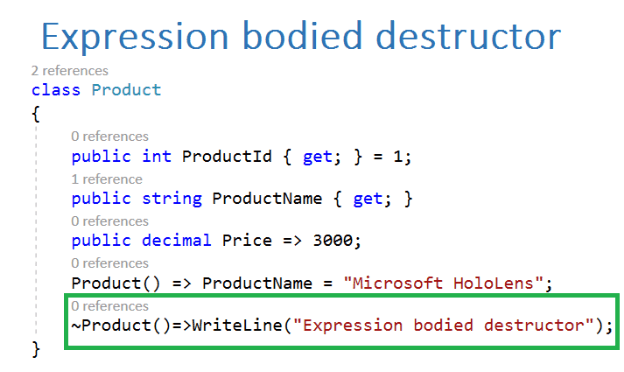Code Snippet
1. class Product {
2.     public int ProductId {
3.         get;
4.     } = 1;
5.     public string ProductName {
6.         get;
7.     }
8.     public decimal Price => 3000;
9.     Product() => ProductName = "Microsoft HoloLens";
10.     ~Product() => WriteLine("Expression bodied destructor");
11. }

Expression bodied getters & settersCode Snippet

1. class Product {
2.     Dictionary < int, decimal > productPriceList = new Dictionary < int, decimal > ();
3.     public int ProductId {
4.         get;
5.         set;
6.     } = 1;
7.     public string ProductName => "Microsoft HoloLens";
8.     public decimal Price {
9.         get => productPriceList[ProductId];
10.         set => productPriceList[ProductId] = value;
11.     }
12. }

It is not necessary to use property inside getter or setter expression. We can also use a variable inside getter and setter. So, the above code can also be written as.

1. class Product {
2.     Dictionary < int, decimal > productPriceList = new Dictionary < int, decimal > ();
3.     public int _productId;
4.     public string ProductName => "Microsoft HoloLens";
5.     public decimal Price {
6.         get => productPriceList[_productId];
7.         set => productPriceList[_productId] = value;
8.     }
9. }

9. Ref returns and locals

Most of the developers must be aware about C# “ref” keyword and its behaviors. As we know that it returns address of the variable rather than value and it can also be used with reference type where new memory allocation has to be done in the calling method. If anyone is not aware about ‘ref’ keyword or wants to brush basic concept of C#, then please go through the article.

Basic Interview Tips in C#

But till C# 6, we can only use ‘ref’ keyword while passing a parameter for method. In C# 7, we can also use ‘ref’ for returning a variable from a method i.e. a method can return variable with reference. We can also store a local variable with reference.

Ref Return

Sample Code

1. int[] x = {
2.     2,
3.     4,
4.     62,
5.     54,
6.     33,
7.     55,
8.     66,
9.     71,
10.     92
11. };
12. public ref int GetFirstOddNumber(int[] numbers) {
13.     for (int i = 0; i < numbers.Length; i++) {
14.         if (numbers[i] % 2 == 1) {
15.             return ref numbers[i];
16.         }
17.     }
18.     throw new Exception("odd number not found");
19. }
20. But there are some restrictions and everything cannot be returned as reference like below code will give error: Dictionary < int, decimal > ProductPriceList = new Dictionary < int, decimal > ();
21. public ref decimal GetProductPriceReference(int productId) {
22.     return ref ProductPriceList[productId];
23. }

Error

CS8156 An expression cannot be used in this context because it may not be returned by reference

Ref locals

As you have seen, in the previous example I am searching for an odd number inside an integer array and if it is not found throwing exception, the method is not returning it as value but as reference. So, you need to store that value also which has been returned as reference. To store it in a local variable, we can use ‘ref’ keyword with local variables, known as ref locals.

Code snippet

1. int[] x = { 2, 4, 62, 54, 33, 55, 66, 71, 92 };
2. ref int oddNum = ref GetFirstOddNumber(x);

Complete Code

1. using static System.Console;
2. namespace RefReturnsAndRefLocals {
3.     class Program {
4.         public ref int GetFirstOddNumber(int[] numbers) {
5.             for (int i = 0; i < numbers.Length; i++) {
6.                 if (numbers[i] % 2 == 1) {
7.                     return ref numbers[i]; //returning as reference
8.                 }
9.             }
10.             throw new Exception("odd number not found");
11.         }
12.         static void Main(string[] args) {
13.             Program p = new Program();
14.             int[] x = {
15.                 2,
16.                 4,
17.                 62,
18.                 54,
19.                 33,
20.                 55,
21.                 66,
22.                 71,
23.                 92
24.             };
25.             ref int oddNum = ref p.GetFirstOddNumber(x); //storing as reference
26.             WriteLine(\$ "\t\t\t\t{oddNum}");
27.             oddNum = 35;
28.             for (int i = 0; i < x.Length; i++) {
29.                 Write(\$ "{x[i]}\t");
30.             }
32.         }
33.     }
34. }

OutputIn the above screenshot, you can see that first time the variable “OddNum” is storing the value 33 with its reference inside the array ‘x’.

If you print “oddNum” first time, then it will print 33 but after that, I have re-assigned its value and set “oddNum =35” now iterating the array and printing elements of array and you can see that whatever I have done, modification for “oddNum” from outside is also reflecting inside the array and internal value has been modified from 33 to 35.

10. Throw Expressions

In C# 7, we can throw an exception directly through expression. Thus, an exception can be thrown from an expression.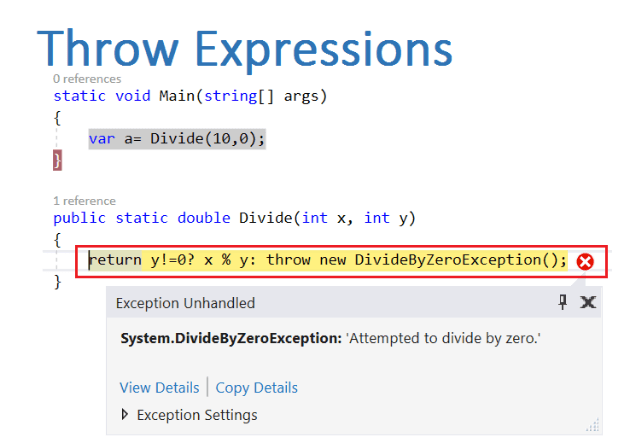Code Snippet

1. class Program {
2.     static void Main(string[] args) {
3.         var a = Divide(10, 0);
4.     }
5.     public static double Divide(int x, int y) {
6.         return y != 0 ? x % y : throw new DivideByZeroException();
7.     }
8. // This is just a sample script. Paste your real code (javascript or HTML) here.
9. if ('this_is' == /an_example/) {
10.     of_beautifier();
11. else {
12.     var a = b ? (c % d) : e[f];
13. }

We can throw any type of exception through the expression like “IndexOutOfRangeException”, “NullReferenceException”, “OutOfMemoryException”, “StackOverflowException” and many other types of exception.

Apart from the above-mentioned features, there are some more features for C# 7, that have been proposed like generalized async (Generalized async return), Replace (Partial Class Enhancements) Records (or Record Types) Non-Nullable Reference Types, Immutable Types etc.

I will discuss these features later on with future release VS 2017.
Next » C# 7 ref And out